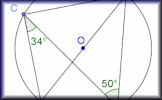#Click on a picture above for a large version and interactive model or show a theorem at Random.

#### Proof of Circle TheoremsArrange the stages of the proofs for the standard circle theorems in the correct order with this drag-and-drop activity.

Transum.org/go/?to=provetheorem

#### Circle Theorem PairsA pairs game based around ten theorems about the angles made with chords, radii and tangents of circles.

Transum.org/go/?to=circletheorempairs

#### Circle Theorem WorksheetOne page of ready-drawn circles for pupils to draw their own diagrams followed by two pages of questions.

Transum.org/go/?to=circletheoremworksheet

#### Circle Theorems ExerciseShow that you understand the circle theorems with this self marking exercise of traditional circle angle questions.

Transum.org/go/?to=circleangles

There are also Circle Theorems Pairs games, a Circle Theorems Worksheet and a Circle Theorems Online Exercise available.

#### Three WaysCan you get to the target number by multiplying together four different one digit numbers? Can you do it in three different ways? There are nine levels to this online challenge and a virtual Transum Trophy available for each level.

This is a Shine+Write visual aid designed to be projected onto a whiteboard for whole class exposition.

You can find other popular visual aids on our Shine+Write page.

Find out about the latests developments on this website by signing up for a Transum Subscription. You will receive a regular newsletter and have access to the answers to most of the puzzles and activities on this site.

Do you have any comments? It is always useful to receive feedback and helps make this free resource even more useful for those learning Mathematics anywhere in the world. Click here to enter your comments.

#### Which Operation?Decide which mathematical operation is required then use it to find the answers. So far this activity has been accessed 218 times and 4 people have earned a Transum Trophy for completing it.For All: